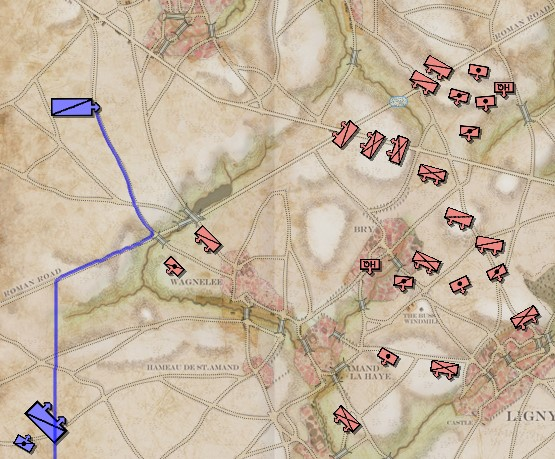# How to rotate sprite to match movement angle?

MonoGame uses radians, right? I thought it did. Anyway, here’s the problem:So, the path of this unit is the blue line. At the end of it’s path, the unit should be facing approximately north, but it’s facing east, instead.

I’m taking the last and the 15th from the last points on the calculated path and using this formula:

``````double angle;
angle = UtilityMethods.CalculateAngle2Radians(tPathList[tPathList.Count-15], tPathList[tPathList.Count - 1]);
TempUnitFacing = angle;
``````

And it calls this method:

``````        const double Rad2Deg = 180.0 / Math.PI;
const double Deg2Rad = Math.PI / 180.0;

/// <summary>
/// Calculates angle in radians between two points and x-axis.
/// </summary>
public static double CalculateAngle2Radians(PointI start, PointI end)
{
return Math.Atan2(start.Y - end.Y, end.X - start.X) * Rad2Deg;
}
``````

But the angle produced is wildly off. It’s not even a case where I can subtract 90 degrees or something.
Anybody have any ideas?

Follow up: I changed this method to:

``````        const double Rad2Deg = 180.0 / Math.PI;
const double Deg2Rad = Math.PI / 180.0;

/// <summary>
/// Calculates angle in radians between two points and x-axis.
/// </summary>
public static double CalculateAngle2Radians(PointI start, PointI end)
{
return Math.Atan2(start.Y - end.Y, end.X - start.X) * Deg2Rad;
}
``````

And the results are always ‘pointing north’.

Thanks!

Math.Atan2 uses radians. In your method you are multiplying by Deg2Rad

You are also calculating start - end, and end - start. They are flipped

It should be

``````        Vector2 directionVector = new Vector2(0, 1);
float radians = (float)Math.Atan2(directionVector.Y, directionVector.X);``````
1 Like

Thank you so much! That’s what I get for googling “C# angle to radians”, grabbing something off of GitHub and not really looking at it. I so miss coding with a partner and having another set of eyes. Thank you again!

1 Like

Nez has some helper math to deal with this stuff… What always gets me is Y is going down. the clockwise and winding conventions to… since we learn trig with Y up + its gets confusng.

``````	/// <summary>
/// returns the angle whose tangent is the quotient (y/x) of two specified numbers
/// </summary>
/// <param name="y">The y coordinate.</param>
/// <param name="x">The x coordinate.</param>
[MethodImpl(MethodImplOptions.AggressiveInlining)]
public static float Atan2(float y, float x)
{
return (float)Math.Atan2(y, x);
}

#endregion

#region Vector2

[MethodImpl(MethodImplOptions.AggressiveInlining)]
public static float AngleBetweenVectors(Vector2 from, Vector2 to)
{
return Atan2(to.Y-from.Y, to.X-from.X);
}

[MethodImpl(MethodImplOptions.AggressiveInlining)]
public static Vector2 AngleToVector(float angleRadians, float length)
{
}
``````

#if GRAPHICS_MG
///

/// helper for moving a value around in a circle.
///

[MethodImpl(MethodImplOptions.AggressiveInlining)]
public static Vector2 RotateAround(Vector2 position, float speed)
{
var time = Time.TotalTime * speed;

``````		var x = Cos(time);
var y = Sin(time);

return new Vector2(position.X + x, position.Y + y);
}
``````

#endif

``````	/// <summary>
/// the rotation is relative to the current position not the total rotation. For example, if you are currently at 90 degrees and
/// want to rotate to 135 degrees, you would use an angle of 45, not 135.
/// </summary>
/// <returns>The around.</returns>
/// <param name="point">Point.</param>
/// <param name="center">Center.</param>
/// <param name="angleInDegrees">Angle in degrees.</param>
[MethodImpl(MethodImplOptions.AggressiveInlining)]
public static Vector2 RotateAround(Vector2 point, Vector2 center, float angleInDegrees)
{
float cos = Cos(angleInDegrees);
float sin = Sin(angleInDegrees);
var rotatedX = cos*(point.X-center.X)-sin*(point.Y-center.Y)+center.X;
var rotatedY = sin*(point.X-center.X)+cos*(point.Y-center.Y)+center.Y;

return new Vector2(rotatedX, rotatedY);
}

/// <summary>
/// the rotation is relative to the current position not the total rotation. For example, if you are currently at 1 Pi radians and
/// want to rotate to 1.5 Pi radians, you would use an angle of 0.5 Pi, not 1.5 Pi.
/// </summary>
/// <returns>The around.</returns>
/// <param name="point">Point.</param>
/// <param name="center">Center.</param>
/// <param name="angleInDegrees">Angle in radians.</param>
[MethodImpl(MethodImplOptions.AggressiveInlining)]
public static Vector2 RotateAroundRadians(Vector2 point, Vector2 center, float angleInRadians)
{
float cos = Cos(angleInRadians);
float sin = Sin(angleInRadians);
var rotatedX = cos*(point.X-center.X)-sin*(point.Y-center.Y)+center.X;
var rotatedY = sin*(point.X-center.X)+cos*(point.Y-center.Y)+center.Y;

return new Vector2(rotatedX, rotatedY);
}``````

Thanks! I’m amazed at how nice people are here on the MonoGame forum (as opposed to the thinly veiled contempt at StackOverflow).

thinly veiled? Stack Exchange is the most gamified , egoist, living in moms basement, post grad but unemployable, anti Einstein, incel -infested rote learning site for ****…never mmind some of the very basic programming questions have half decent answers … one gjy was trying to link to a c lib to c# to normalize some vectors. i suggested porting the c lib to c# and using numerics lib and SIMD and compex numbers not using the c lib and arrays… Downvoted. -10 for not answering the dll import question exactly. on Natural philosophy and physics, downvoted for totally relevant ontological points in 4D cosmology , quoting Eisntein adn Hawking in a sort of discussion (there are no right answers or socratic dialogs unless u got tonsof points) dont ever contribute to them. they might have an answer or two but old and not open to new approches, presence of females, wild or new ideas that are relevant but the downvoters lack the imagination to make the connection. Einstein or Hawking would have like -666 score in physcs on there, if he was still “moving”. tI think we all miss coding with other some eyes, preferably in the room, covid screwed that up…sorry for the rant its summer, venting frustration at being at computer, behind schedule.

Uh, yeah. That’s what I meant.

1 Like